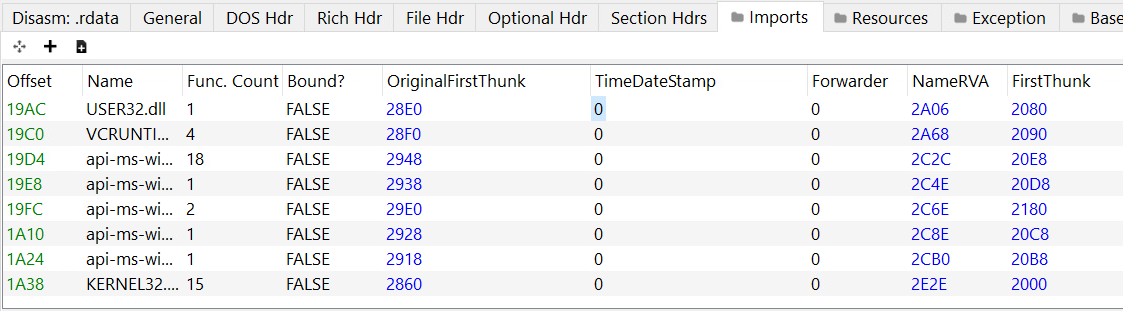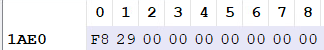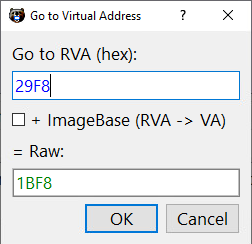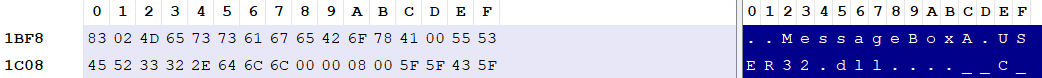A dive into the PE file format - PE file structure - Part 5: PE Imports (Import Directory Table, ILT, IAT)

A dive into the PE file format - PE file structure - Part 5: PE Imports (Import Directory Table, ILT, IAT)

Introduction

In this post we’re going to talk about a very important aspect of PE files, the PE imports. To understand how PE files handle their imports, we’ll go over some of the Data Directories present in the Import Data section (.idata), the Import Directory Table, the Import Lookup Table (ILT) or also referred to as the Import Name Table (INT) and the Import Address Table (IAT).

Import Directory Table

The Import Directory Table is a Data Directory located at the beginning of the .idata section.

It consists of an array of IMAGE_IMPORT_DESCRIPTOR structures, each one of them is for a DLL.
It doesn’t have a fixed size, so the last IMAGE_IMPORT_DESCRIPTOR of the array is zeroed-out (NULL-Padded) to indicate the end of the Import Directory Table.

IMAGE_IMPORT_DESCRIPTOR is defined as follows:

typedef struct _IMAGE_IMPORT_DESCRIPTOR {
union {
DWORD   Characteristics;
DWORD   OriginalFirstThunk;
} DUMMYUNIONNAME;
DWORD   TimeDateStamp;
DWORD   ForwarderChain;
DWORD   Name;
DWORD   FirstThunk;
} IMAGE_IMPORT_DESCRIPTOR;
typedef IMAGE_IMPORT_DESCRIPTOR UNALIGNED *PIMAGE_IMPORT_DESCRIPTOR;
• OriginalFirstThunk: RVA of the ILT.
• TimeDateStamp: A time date stamp, that’s initially set to 0 if not bound and set to -1 if bound.
In case of an unbound import the time date stamp gets updated to the time date stamp of the DLL after the image is bound.
In case of a bound import it stays set to -1 and the real time date stamp of the DLL can be found in the Bound Import Directory Table in the corresponding IMAGE_BOUND_IMPORT_DESCRIPTOR .
We’ll discuss bound imports in the next section.
• ForwarderChain: The index of the first forwarder chain reference.
This is something responsible for DLL forwarding. (DLL forwarding is when a DLL forwards some of its exported functions to another DLL.)
• Name: An RVA of an ASCII string that contains the name of the imported DLL.
• FirstThunk: RVA of the IAT.

Bound Imports

A bound import essentially means that the import table contains fixed addresses for the imported functions.
These addresses are calculated and written during compile time by the linker.

Using bound imports is a speed optimization, it reduces the time needed by the loader to resolve function addresses and fill the IAT, however if at run-time the bound addresses do not match the real ones then the loader will have to resolve these addresses again and fix the IAT.

When discussing IMAGE_IMPORT_DESCRIPTOR.TimeDateStamp, I mentioned that in case of a bound import, the time date stamp is set to -1 and the real time date stamp of the DLL can be found in the corresponding IMAGE_BOUND_IMPORT_DESCRIPTOR in the Bound Import Data Directory.

Bound Import Data Directory

The Bound Import Data Directory is similar to the Import Directory Table, however as the name suggests, it holds information about the bound imports.

It consists of an array of IMAGE_BOUND_IMPORT_DESCRIPTOR structures, and ends with a zeroed-out IMAGE_BOUND_IMPORT_DESCRIPTOR.

IMAGE_BOUND_IMPORT_DESCRIPTOR is defined as follows:

typedef struct _IMAGE_BOUND_IMPORT_DESCRIPTOR {
DWORD   TimeDateStamp;
WORD    OffsetModuleName;
WORD    NumberOfModuleForwarderRefs;
// Array of zero or more IMAGE_BOUND_FORWARDER_REF follows
} IMAGE_BOUND_IMPORT_DESCRIPTOR,  *PIMAGE_BOUND_IMPORT_DESCRIPTOR;
• TimeDateStamp: The time date stamp of the imported DLL.
• OffsetModuleName: An offset to a string with the name of the imported DLL.
It’s an offset from the first IMAGE_BOUND_IMPORT_DESCRIPTOR
• NumberOfModuleForwarderRefs: The number of the IMAGE_BOUND_FORWARDER_REF structures that immediately follow this structure.
IMAGE_BOUND_FORWARDER_REF is a structure that’s identical to IMAGE_BOUND_IMPORT_DESCRIPTOR, the only difference is that the last member is reserved.

That’s all we need to know about bound imports.

Import Lookup Table (ILT)

Sometimes people refer to it as the Import Name Table (INT).

Every imported DLL has an Import Lookup Table.
IMAGE_IMPORT_DESCRIPTOR.OriginalFirstThunk holds the RVA of the ILT of the corresponding DLL.

The ILT is essentially a table of names or references, it tells the loader which functions are needed from the imported DLL.

The ILT consists of an array of 32-bit numbers (for PE32) or 64-bit numbers for (PE32+), the last one is zeroed-out to indicate the end of the ILT.

Each entry of these entries encodes information as follows:

• Bit 31/63 (most significant bit): This is called the Ordinal/Name flag, it specifies whether to import the function by name or by ordinal.
• Bits 15-0: If the Ordinal/Name flag is set to 1 these bits are used to hold the 16-bit ordinal number that will be used to import the function, bits 30-15/62-15 for PE32/PE32+ must be set to 0.
• Bits 30-0: If the Ordinal/Name flag is set to 0 these bits are used to hold an RVA of a Hint/Name table.

Hint/Name Table

A Hint/Name table is a structure defined in winnt.h as IMAGE_IMPORT_BY_NAME:

typedef struct _IMAGE_IMPORT_BY_NAME {
WORD    Hint;
CHAR   Name;
} IMAGE_IMPORT_BY_NAME, *PIMAGE_IMPORT_BY_NAME;
• Hint: A word that contains a number, this number is used to look-up the function, that number is first used as an index into the export name pointer table, if that initial check fails a binary search is performed on the DLL’s export name pointer table.
• Name: A null-terminated string that contains the name of the function to import.

On disk, the IAT is identical to the ILT, however during bounding when the binary is being loaded into memory, the entries of the IAT get overwritten with the addresses of the functions that are being imported.

Summary

So to summarize what we discussed in this post, for every DLL the executable is loading functions from, there will be an IMAGE_IMPORT_DESCRIPTOR within the Image Directory Table.
The IMAGE_IMPORT_DESCRIPTOR will contain the name of the DLL, and two fields holding RVAs of the ILT and the IAT.
The ILT will contain references for all the functions that are being imported from the DLL.
The IAT will be identical to the ILT until the executable is loaded in memory, then the loader will fill the IAT with the actual addresses of the imported functions.
If the DLL import is a bound import, then the import information will be contained in IMAGE_BOUND_IMPORT_DESCRIPTOR structures in a separate Data Directory called the Bound Import Data Directory.

Let’s take a quick look at the import information inside of an actual PE file.

Here’s the Import Directory Table of the executable:All of these entries are IMAGE_IMPORT_DESCRIPTORs.

As you can see, the TimeDateStamp of all the imports is set to 0, meaning that none of these imports are bound, this is also confirmed in the Bound? column added by PE-bear.

For example, if we take USER32.dll and follow the RVA of its ILT (referenced by OriginalFirstThunk), we’ll find only 1 entry (because only one function is imported), and that entry looks like this:This is a 64-bit executable, so the entry is 64 bits long.
As you can see, the last byte is set to 0, indicating that a Hint/Table name should be used to look-up the function.
We know that the RVA of this Hint/Table name should be referenced by the first 2 bytes, so we should follow RVA 0x29F8:Now we’re looking at an IMAGE_IMPORT_BY_NAME structure, first two bytes hold the hint, which in this case is 0x283, the rest of the structure holds the full name of the function which is MessageBoxA.
We can verify that our interpretation of the data is correct by looking at how PE-bear parsed it, and we’ll see the same results:Conclusion

That’s all I have to say about PE imports, in the next post I’ll discuss PE base relocations.